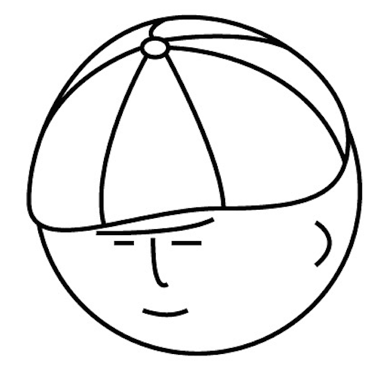# マツシタのお勉強メモ

#### マツシタのお勉強

##### welcome to my engineering blog
###### Hacker Rank# Count Path Sum in Binary Tree

Problem You are given a binary tree in which each node contains an integer value. Find the number of paths that sum to a given value. The path does not need to start or end at the root or a leaf, but it must go downwards (traveling only fr…

# Print all paths which sum to a given value in a Binary Tree

Problem You are given a binary tree in which each node contains a value. Design an algorithm to print all paths which sum to a given value. The path dose not need to start or end at the root or leaf. Solution First, let’s approach this pro…